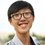# $a+b=b+a$? Not quite.

Try to construct two different programs to calculate the value of

$\sum_{k=1}^\infty \frac{1}{k^2}$

Some will immediately noticed that this is actually $\frac{\pi^2}{6}$, but we try doing it by definition.

First, we calculate the sum by usual order

$1+\frac{1}{4}+\dots+\frac{1}{(N-1)^2}+\frac{1}{N^2}$

Next, we calculate the sum by reversed order

$\frac{1}{N^2}+\frac{1}{(N-1)^2}+\dots+\frac{1}{4}+1$

The answer should be the same, even for computers, right? However, when I tried in C++

First approach:

 1 2 3 4 5 6 7 float zeta_up(long n){ float ans = 0.; for (long k=1; k<=n; k++){ ans += 1./float(k)/float(k); } return ans; } 

Second approach:

 1 2 3 4 5 6 7 float zeta_down(long n){ float ans = 0.; for (long k=n; k>=1; k--){ ans += 1./float(k)/float(k); } return ans; } 

And a constant value for reference:

 1 const float zeta = M_PI*M_PI/6.; 

Three values are:

 1 2 3 zeta_up = 1.64473 zeta_down = 1.64493 constant = 1.64493 

So, the second approach seems more accurate, why is that?

The discrepancy is due to roundoff issues. A float holds only so many digits and (on my computer) the epsilon value for a float is around $10^{-7}$. This means that by the time the sum reaches $k = 106$, the value held in ans is too large for the addition of $10^{-12}$ to have any effect. Many terms at the end of the series have little or no effect on the sum; their contribution is lost. However, those tail terms do have a cumulative contribution on the actual sum and are needed to give an accurate result. By summing in the reverse order these small terms do not have their contributions lost.

C++ For Mathematicians by Edward ScheinermanNote by Christopher Boo
6 years, 8 months ago

This discussion board is a place to discuss our Daily Challenges and the math and science related to those challenges. Explanations are more than just a solution — they should explain the steps and thinking strategies that you used to obtain the solution. Comments should further the discussion of math and science.

When posting on Brilliant:

• Use the emojis to react to an explanation, whether you're congratulating a job well done , or just really confused .
• Ask specific questions about the challenge or the steps in somebody's explanation. Well-posed questions can add a lot to the discussion, but posting "I don't understand!" doesn't help anyone.
• Try to contribute something new to the discussion, whether it is an extension, generalization or other idea related to the challenge.
• Stay on topic — we're all here to learn more about math and science, not to hear about your favorite get-rich-quick scheme or current world events.

MarkdownAppears as
*italics* or _italics_ italics
**bold** or __bold__ bold
- bulleted- list
• bulleted
• list
1. numbered2. list
1. numbered
2. list
Note: you must add a full line of space before and after lists for them to show up correctly
paragraph 1paragraph 2

paragraph 1

paragraph 2

[example link](https://brilliant.org)example link
> This is a quote
This is a quote
    # I indented these lines
# 4 spaces, and now they show
# up as a code block.

print "hello world"
# I indented these lines
# 4 spaces, and now they show
# up as a code block.

print "hello world"
MathAppears as
Remember to wrap math in $$ ... $$ or $ ... $ to ensure proper formatting.
2 \times 3 $2 \times 3$
2^{34} $2^{34}$
a_{i-1} $a_{i-1}$
\frac{2}{3} $\frac{2}{3}$
\sqrt{2} $\sqrt{2}$
\sum_{i=1}^3 $\sum_{i=1}^3$
\sin \theta $\sin \theta$
\boxed{123} $\boxed{123}$

## Comments

Sort by:

Top Newest

At first I thought this was something like @Mursalin Habib 's fallacies. After I finished reading it I realized it was a computer science problem :D

- 6 years, 8 months ago

Log in to reply

Try doing the same using a double instead of a float, does it return a similar result?

- 6 years, 8 months ago

Log in to reply

When written in Visual Basic, I did not face this problem at all.

- 6 years, 8 months ago

Log in to reply

Can you explain why Visual Basic worked better than C++?

Staff - 6 years, 8 months ago

Log in to reply

Possibly because I used double precision variables (8 bytes) not float which is single precision (4 bytes) so the machine epsilon in my case is much smaller.

- 6 years, 8 months ago

Log in to reply

Java has BigDecimal. I got

1.6449330668487264363057484999793918558856165440796 1.6449330668487264363057484999793918558856165440640 1.6449340668482264

Using MathContext mc = new MathContext(50, RoundingMode.HALF_UP); n=1000000

Executes in a couple of minutes.

My 2 numbers deviate around the 48th decimal place.

But my constant, deviates around the 6th decimal place.

If anyone wants to see the entire source code, let me know.

- 6 years, 8 months ago

Log in to reply

Do you have small file to send an accessible Java BigDecimal program via the internet? Where can I obtain instead?

- 5 years, 8 months ago

Log in to reply

"zeta"? Uhm. Where is the zeta?

- 6 years, 8 months ago

Log in to reply

constant is the zeta

- 6 years, 8 months ago

Log in to reply

the problem is the method of storing number (float & double ) specially float.

- 6 years, 6 months ago

Log in to reply

This is just a sum of Fourier Series found in engineering mathematics for its exact proof. Sum to computing's significant figures always incur serious errors for series of less convergence which must go for a long run of up to 10^12 terms. Product to computing is relatively much more favorable with less inaccuracies. Turbo Pascal's EXTENDED of 18 S.F. appears to user is quite good to replace REAL by {\$N+} or adjust numeric processing from software to 8087/80287 at Compiler under Options.

- 5 years, 8 months ago

Log in to reply

programming

- 6 years, 7 months ago

Log in to reply

×

Problem Loading...

Note Loading...

Set Loading...